# How to choose a suitable Transistor in a circuit for amplification

#### Walt Peter

Joined Mar 18, 2016
5
Hello. I am now creating my first project. I faced a problem with transistor's types. I originally using transistor S 2013 for my circuit with Collector 9 volts and Base 9 volts. The transistor heated up and failed to function anymore. So, what should I do? Should I replace the transistors (For example transistor 2 N 2222)? And, since I putted the transistors in amplifier mode, if I put 9 volts to Collector and Base respectively, can I get a 18 volts output at Emitter? Thank you.
Peter.

#### Dodgydave

Joined Jun 22, 2012
11,151
Transistors use 0.7v base/emmiter voltage, so any more and it will blow, Can you post the circuit please.?

#### Walt Peter

Joined Mar 18, 2016
5
Can you post the circuit please.?
Oh. Sorry. Here is the circuit. I just create the circuit. Thank you.

#### dl324

Joined Mar 30, 2015
16,206
You have the transistor connected backwards. If you applied power, it's probably dead. You need a current limiting resistor on the base.

You also need a snubber circuit to protect the transistor from back EMF.

•Walt Peter

#### Dodgydave

Joined Jun 22, 2012
11,151
•Walt Peter

#### Walt Peter

Joined Mar 18, 2016
5
Does this OK? I mean the parts, especially the transistors. Thank you.#### dl324

Joined Mar 30, 2015
16,206
Does this OK?
Are you just trying to turn the motor on? Or are you trying to control speed and direction?

The circuit you have can work, but you need snubber diodes and a controller to drive the transistors.

#### Walt Peter

Joined Mar 18, 2016
5
Are you just trying to turn the motor on? Or are you trying to control speed and direction?

The circuit you have can work, but you need snubber diodes and a controller to drive the transistors.
I tried to control the speed and direction of the DC Motor. Thank you.

#### bertus

Joined Apr 5, 2008
22,233
Hello,

Why are the emitters of Q3 and Q4 connected to a separate ground?
There is no current path for the transistors to drive them.

Bertus

#### hobbyist

Joined Aug 10, 2008
892
Hello, lets do some basic circuit analysis of your proposal.

Start at the positive side of power source, and trace the path to the neg. side of source, first through Q1, the base emitter diode of the PNP transistor Q1 is forward biased, as pos. flow enters the emitter and exits out the base of Q1. Also the same path of flow goes through the branch containing the base emitter diode of Q2, as wel.

So far you have two PNP transistors forward biased at there bases.

Now lets analyse the path of current through the collector terminals of Q1, and Q2.
There is no positive flow that can go from the collectors of Q1, and Q2, to the neg. side of source because two conditions are keeping Q3 and Q4 at cutoff.

First the base of Q3 and Q4 need to be positive with respect to there emitters since they are NPN transistors. In the schematic Q3 and Q4, have neg. side of source connected to there bases.

Also the emitters of Q3, and Q4 need to be connected to the neg. side of source.

Assuming the ground symbol was attached to the neg. side of source as well as the emitters then the emitters would have the neg. potential needed.

So the emitters are biased properly under the above assumption, but the bases are reversed biased.

So under a test of your circuit performance, you could not drive the load in the bridge configuration, because there is no current flow through the load.

Here is an example of one of several different ways this could be fixed to work properly.

Whenever you design a transistor circuit that works in a switching mode, try to get into a habit of drawing it up using switches first, to take the place of transistors when you start your design.

In this example pic. draw up the function of a two directional (forward and reverse) motor running circuit.
And put switches in places that would make the motor function in forward and reverse mode.

Notice in this classic bridge driver circuit, that the switches labeled (1) and (2) are together as pairs top and bottom. Oppositie corners of eachother diagonal to eachother. These diagonal pairs form a bridge circuit through the load., in both directions.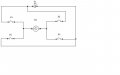Now analyse the circuit topology by drawing in the load current flows, for both directions of running the motor.

forward direction: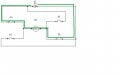reverse direction: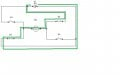Then after your satisfied that the circuit will work properly using these switches then put the transistors in place of the switches.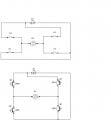now draw in what you want the transistors to do to take the place of the switches.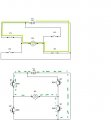then design the circuitry needed to turn these transistors on to make them do what there suppose to do.
Here you see the bias paths of current with the resistors at the bases of the transistors, shown in red lines to Q1 NPN, and Q1 PNP.

forward direction: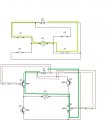reverse direction: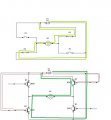Now that you have the topology worked out, its now time to calculate the values of the components needed to design this circuit.
First remove all the inputs, to the battery and substitute input symbols, because these inputs could be manual switches or electronic signals, so now they are considered input signals only.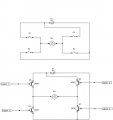First assume a transistor acting as a switch will be in saturation, therefor a value of around 0.2V will be dropped across the transistors from collector to emitter, (this assumption is for calculation purposes).

(Now you are using a 100 ohm resistive load in your scheme, so I'll use that value for the motor.)

Now you do some preliminary experimenting and through test and measurements, you find that around 100 ohms impedance seems to be good for your motor at top speed that you desire to run the motor at for this design.

Now its time to calculate the bias resistor needed for the transistor to turn on and produce the current you calculated needed to be for the motor to run optimal.

using kirchoffs voltage laws calculate the voltage drops in one circuit path from positive. to negative terminals of the power source,,. ( or the other way around) which ever your comfortable with.

Here is the voltage loop through transistor Q2 NPN. base emitter junction.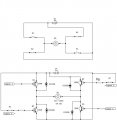Loop equation [ +9v - VRB - Vbe = 0v ]
another assumption is to use a value of around 0.6v to 0.8v for the voltage drop across the base emitter diode, so using 0.7v is adequate enough.

Rewritting the equation now becomes [ +9v - VRB - 0.7v = 0v ] Therefor VRB = 9v - 0.7v = 8.3v

Now voltage drop across RB has been calculated we now need to move onto calculating the base current that will flow through this resistor in order to solve for the value of this resistor.

To do that another voltage loop needs to be analysed, And that is the drops across both Q2 NPN, and Q2 PNP trasnsitors (which form the bridge circuit to turn the motor on in one of its directions), so as to solve for the voltage drop across the load given as 100 ohms impedance.

Here is the equation to solve for Voltage drop across the load [Vload] Remember assuming 0.2v across a transistor in this mode.

equation loop { +9v - VEC Q2 PNP - VM1 - VCE Q2 NPN = 0 }

{ +9v - 0.2v - VM1 - 0.2v = 0 } therefor volt drop across the motor M1 is (9v - 0.4v = 8.6v) = VM1.

Now Vm1 is calculated so now next is to solve for the current through this load M1.

Vm1 / 100 ohms = 8.6v / 100 ohms = .086 A or 86mA.

Ok finally to solve for the value of the base bias resistors use the calculated values and the assumptions given earlier.

One more assumption is made for base current used to saturate a transistor to make it act as a switch. Take the collector current and divide it by 10 for the base current value.

therefor if the load current through the motor was calculated to be 86mA, and if we follow that current flow through its path we find that it will flow directly through both complementary pairs of transistors Q2 pair. So now we know that the full load current flows through both Q2's so that makes the collector current of the transistors equal the load current of 86mA.

So 86mA divided by 10 will be the calculated base current needed for these transistors to turn on as strong as we designed the circuit to perform at.

That base current is 8.6mA, so now we can solve for the value of RB by taking the voltage drop across RB (VRB) and divide it by this base current value of 8.6mA to give around 965 ohms.

The nearest nominal resistor could be 1K ohms or 910 ohms. Lets choose 910 ohms to ensure a heavy base current.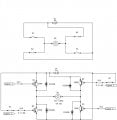Ok thats not all, we need to make sure a 1/4 wt. res will be satisfactory for this design.
So using the power formula I^2 * R makes the power used in the 910 ohm resistance be around 67 mw. perfectly fine. power across the transistor would be power formula (E * I) = (0.2v * 86mA) = 17 mw.

Now one more thing to consider when designing for an inductive load, when a motor stops running there is the collapse of the magnetic field causing what is commonly known as inductive kickback, and this will blow out the transistors as current is trying to flow heavily in reverse bias across the CE junctions of the transistors, causing them to fail, so thats why I have those diodes across the CE terminals of each transistor, note that these diode are in the reverse of the CE diode directions,
under normal transistor action of current flow, the diodes are reversed biased into cutoff, but when the transitors are shut down due to stopping the motor from running or reverse direction of the motor, the inductive kickback current will flow through the diodes in forward bias direction while at the same time reverse biasing the CE of the transistors, these diodes allow kickback current to flow around the transistors so as to not destroy the transistors with reverse current flow.

So maybe this could be of some little help in understanding your design.
Have fun working with transistors they are fun to design circuits with.

#### David.uulf

Joined Mar 25, 2017
1
Hello, lets do some basic circuit analysis of your proposal.
wow that was one of the best systematic work throughs i've seen after reading that i feel like i have some skills to start working something like this out on my own (with references back to this). i love that instead of just giving the answer you gave a way to work it out for ourselves and in a way that we could adapt it to a number of other applications.
thanks so much for the effort you put in to make that answer. i learnt so much.
regards
dave

•hobbyist

#### hobbyist

Joined Aug 10, 2008
892
wow that was one of the best systematic work throughs i've seen after reading that i feel like i have some skills to start working something like this out on my own (with references back to this). i love that instead of just giving the answer you gave a way to work it out for ourselves and in a way that we could adapt it to a number of other applications.
thanks so much for the effort you put in to make that answer. i learnt so much.
regards
dave
Thank you for letting me know how much it has helped you, I'm always trying to share my learning experience in this at the hobby level, for those who are just starting out in the basics of electronic circuit design.

#### Mellisa_K

Joined Apr 2, 2017
391
Hello, lets do some basic circuit analysis of your proposal.

Start at the positive side of power source, and trace the path to the neg. side of source, first through Q1, the base emitter diode of the PNP transistor Q1 is forward biased, as pos. flow enters the emitter and exits out the base of Q1. Also the same path of flow goes through the branch containing the base emitter diode of Q2, as wel.

So far you have two PNP transistors forward biased at there bases.

Now lets analyse the path of current through the collector terminals of Q1, and Q2.
There is no positive flow that can go from the collectors of Q1, and Q2, to the neg. side of source because two conditions are keeping Q3 and Q4 at cutoff.

First the base of Q3 and Q4 need to be positive with respect to there emitters since they are NPN transistors. In the schematic Q3 and Q4, have neg. side of source connected to there bases.

Also the emitters of Q3, and Q4 need to be connected to the neg. side of source.

Assuming the ground symbol was attached to the neg. side of source as well as the emitters then the emitters would have the neg. potential needed.

So the emitters are biased properly under the above assumption, but the bases are reversed biased.

So under a test of your circuit performance, you could not drive the load in the bridge configuration, because there is no current flow through the load.

Here is an example of one of several different ways this could be fixed to work properly.

Whenever you design a transistor circuit that works in a switching mode, try to get into a habit of drawing it up using switches first, to take the place of transistors when you start your design.

In this example pic. draw up the function of a two directional (forward and reverse) motor running circuit.
And put switches in places that would make the motor function in forward and reverse mode.

Notice in this classic bridge driver circuit, that the switches labeled (1) and (2) are together as pairs top and bottom. Oppositie corners of eachother diagonal to eachother. These diagonal pairs form a bridge circuit through the load., in both directions.

View attachment 102669

Now analyse the circuit topology by drawing in the load current flows, for both directions of running the motor.

forward direction:

View attachment 102670

reverse direction:

View attachment 102671

Then after your satisfied that the circuit will work properly using these switches then put the transistors in place of the switches.

View attachment 102672

now draw in what you want the transistors to do to take the place of the switches.

View attachment 102673

then design the circuitry needed to turn these transistors on to make them do what there suppose to do.
Here you see the bias paths of current with the resistors at the bases of the transistors, shown in red lines to Q1 NPN, and Q1 PNP.

forward direction:

View attachment 102674

reverse direction:

View attachment 102675

Now that you have the topology worked out, its now time to calculate the values of the components needed to design this circuit.
First remove all the inputs, to the battery and substitute input symbols, because these inputs could be manual switches or electronic signals, so now they are considered input signals only.

View attachment 102677

First assume a transistor acting as a switch will be in saturation, therefor a value of around 0.2V will be dropped across the transistors from collector to emitter, (this assumption is for calculation purposes).

(Now you are using a 100 ohm resistive load in your scheme, so I'll use that value for the motor.)

Now you do some preliminary experimenting and through test and measurements, you find that around 100 ohms impedance seems to be good for your motor at top speed that you desire to run the motor at for this design.

Now its time to calculate the bias resistor needed for the transistor to turn on and produce the current you calculated needed to be for the motor to run optimal.

using kirchoffs voltage laws calculate the voltage drops in one circuit path from positive. to negative terminals of the power source,,. ( or the other way around) which ever your comfortable with.

Here is the voltage loop through transistor Q2 NPN. base emitter junction.

View attachment 102676

Loop equation [ +9v - VRB - Vbe = 0v ]
another assumption is to use a value of around 0.6v to 0.8v for the voltage drop across the base emitter diode, so using 0.7v is adequate enough.

Rewritting the equation now becomes [ +9v - VRB - 0.7v = 0v ] Therefor VRB = 9v - 0.7v = 8.3v

Now voltage drop across RB has been calculated we now need to move onto calculating the base current that will flow through this resistor in order to solve for the value of this resistor.

To do that another voltage loop needs to be analysed, And that is the drops across both Q2 NPN, and Q2 PNP trasnsitors (which form the bridge circuit to turn the motor on in one of its directions), so as to solve for the voltage drop across the load given as 100 ohms impedance.

Here is the equation to solve for Voltage drop across the load [Vload] Remember assuming 0.2v across a transistor in this mode.

equation loop { +9v - VEC Q2 PNP - VM1 - VCE Q2 NPN = 0 }

{ +9v - 0.2v - VM1 - 0.2v = 0 } therefor volt drop across the motor M1 is (9v - 0.4v = 8.6v) = VM1.

Now Vm1 is calculated so now next is to solve for the current through this load M1.

Vm1 / 100 ohms = 8.6v / 100 ohms = .086 A or 86mA.

Ok finally to solve for the value of the base bias resistors use the calculated values and the assumptions given earlier.

One more assumption is made for base current used to saturate a transistor to make it act as a switch. Take the collector current and divide it by 10 for the base current value.

therefor if the load current through the motor was calculated to be 86mA, and if we follow that current flow through its path we find that it will flow directly through both complementary pairs of transistors Q2 pair. So now we know that the full load current flows through both Q2's so that makes the collector current of the transistors equal the load current of 86mA.

So 86mA divided by 10 will be the calculated base current needed for these transistors to turn on as strong as we designed the circuit to perform at.

That base current is 8.6mA, so now we can solve for the value of RB by taking the voltage drop across RB (VRB) and divide it by this base current value of 8.6mA to give around 965 ohms.

The nearest nominal resistor could be 1K ohms or 910 ohms. Lets choose 910 ohms to ensure a heavy base current.

View attachment 102678

Ok thats not all, we need to make sure a 1/4 wt. res will be satisfactory for this design.
So using the power formula I^2 * R makes the power used in the 910 ohm resistance be around 67 mw. perfectly fine. power across the transistor would be power formula (E * I) = (0.2v * 86mA) = 17 mw.

Now one more thing to consider when designing for an inductive load, when a motor stops running there is the collapse of the magnetic field causing what is commonly known as inductive kickback, and this will blow out the transistors as current is trying to flow heavily in reverse bias across the CE junctions of the transistors, causing them to fail, so thats why I have those diodes across the CE terminals of each transistor, note that these diode are in the reverse of the CE diode directions,
under normal transistor action of current flow, the diodes are reversed biased into cutoff, but when the transitors are shut down due to stopping the motor from running or reverse direction of the motor, the inductive kickback current will flow through the diodes in forward bias direction while at the same time reverse biasing the CE of the transistors, these diodes allow kickback current to flow around the transistors so as to not destroy the transistors with reverse current flow.

So maybe this could be of some little help in understanding your design.
Have fun working with transistors they are fun to design circuits with.
Incrediblely generous post hobbyist! Great help for my learning. Iwill print that and keep it in my "transistor" file. Thankyou

•hobbyist

#### hobbyist

Joined Aug 10, 2008
892
Incrediblely generous post hobbyist! Great help for my learning. Iwill print that and keep it in my "transistor" file. Thankyou
I'm glad this post is very helpful, I really enjoy sharing electronic circuit design from my experience, at the hobby level.

•Mellisa_K

#### Mellisa_K

Joined Apr 2, 2017
391
I'm glad this post is very helpful, I really enjoy sharing electronic circuit design from my experience, at the hobby level.
Yes indeed I am a new member and I am using these forums to learn about my new hobby. I think I am very fortunate to be the beneficiary of the wisdom of so many experienced people who are so willing to help. Viva AAC!

#### hobbyist

Joined Aug 10, 2008
892
Yes indeed I am a new member and I am using these forums to learn about my new hobby. I think I am very fortunate to be the beneficiary of the wisdom of so many experienced people who are so willing to help. Viva AAC!
I do some blogs on this forum, to share how to design basic transistor circuits, I try to share all the steps in the design process, so a beginner can buld these circuits with success.
Here is an example blog of how to design step by step a Transistor LED bargraph.
I have many more on my blogs, if your interested in basic circuit design at the hobby level.

here is a link to this blog.
•